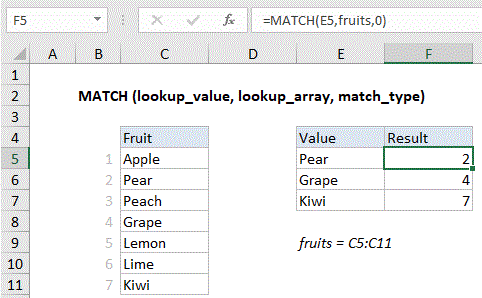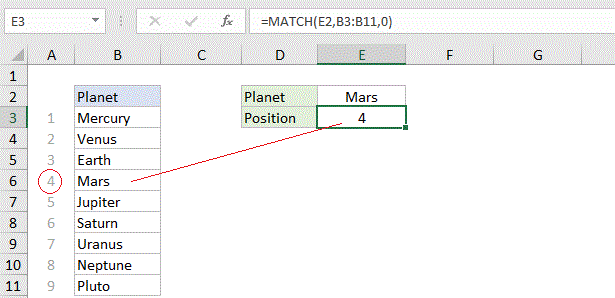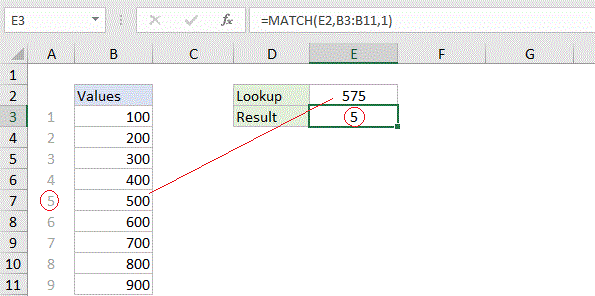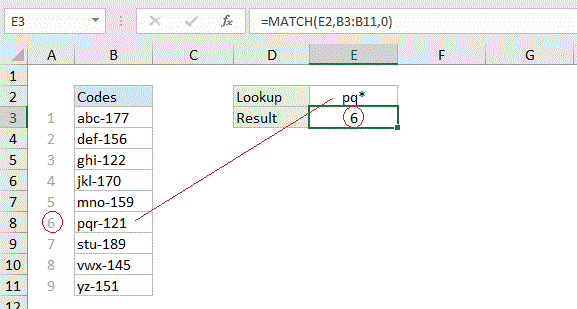## Excel Office

Excel How Tos, Tutorials, Tips & Tricks, Shortcuts

# How to use Excel MATCH Function

This Excel tutorial explains how to use the MATCH function with syntax and examples.

## Excel MATCH function Description

MATCH is an Excel function used to locate the position of a lookup value in a row, column, or table. MATCH supports approximate and exact matching, and wildcards (* ?) for partial matches. Often, the INDEX function is combined with MATCH to retrieve the value at the position returned by MATCH.#### Basic exact match exampleExplanation: When match type is set to zero, MATCH performs an exact match. In the example above; the formula in E3 is:  MATCH(E2,B3:B11,)

### Return

A number representing a position in lookup_array.

### Syntax

MATCH (lookup_value, lookup_array, [match_type])
Match offers several different matching modes, which makes it more flexible than other lookup functions. Used together with INDEX, MATCH can retrieve the value at the matched position.

Note: Match type information

• If match_type is 1, MATCH finds the largest value that is less than or equal to lookup_value. The lookup_array must be sorted in ascending order.
• If match_type is 0, MATCH finds the first value exactly equal to lookup_valuelookup_array does not need to be sorted.
• If match_type is -1, MATCH finds the smallest value that is greater than or equal to lookup_value. The lookup_array must be sorted in descending order.
• If match_type is omitted, it is assumed to be 1.
• Note: All match types will find an exact match.
Also See:   Find closest match in Excel

###Explanation: When match type is set to 1, MATCH will perform an approximate match on values sorted A-Z, finding the largest value less than or equal to the lookup value. In the example shown below, the formula in E3 is:

### Basic wildcard matchExplanation: When match type is set to zero, MATCH can perform a match using wildcards. In the example shown below, the formula in E3 is: MATCH(E2,B3:B11,)

This is equivalent to:

`MATCH("pq*",B3:B11,)`
Also See:   Find missing values in Excel